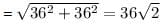NEET  >  Test: Motion in a Plane

# Test: Motion in a Plane

Test Description

## 5 Questions MCQ Test Physics Class 11 | Test: Motion in a Plane

Test: Motion in a Plane for NEET 2023 is part of Physics Class 11 preparation. The Test: Motion in a Plane questions and answers have been prepared according to the NEET exam syllabus.The Test: Motion in a Plane MCQs are made for NEET 2023 Exam. Find important definitions, questions, notes, meanings, examples, exercises, MCQs and online tests for Test: Motion in a Plane below.
Solutions of Test: Motion in a Plane questions in English are available as part of our Physics Class 11 for NEET & Test: Motion in a Plane solutions in Hindi for Physics Class 11 course. Download more important topics, notes, lectures and mock test series for NEET Exam by signing up for free. Attempt Test: Motion in a Plane | 5 questions in 10 minutes | Mock test for NEET preparation | Free important questions MCQ to study Physics Class 11 for NEET Exam | Download free PDF with solutions
 1 Crore+ students have signed up on EduRev. Have you?
Test: Motion in a Plane - Question 1

### A stone is thrown upwards with initial velocity of 20 m s-1, the height that stone will reach would be:

Detailed Solution for Test: Motion in a Plane - Question 1

Initial velocity = u = 20 m/s
Final velocity = v = 0     ( at maximum height , v = 0 )
Acceleration due to gravity(g)  in this case, is taken as negative.
This is because when the direction of motion of object is opposite to "g" , then value of g is taken as -ve.
Hence, g = -9.8 m/s²
Using v2 - u2 = 2gh
02 - 202 = 2 * (- 9.8) * h
- 400 = -19.6 h
h = - 400 /(-19.6) = 20.408 m (approximately)

Test: Motion in a Plane - Question 2

### A particle has an initial velocity of 3i + 4j and an acceleration of 0.4i + 0.3j. Its speed after 10s is:

Detailed Solution for Test: Motion in a Plane - Question 2

Use equation of kinematics: v = u + at
Initial velocity, u = 3i + 4j
Acceleration, a = 0.4i + 0.3j
Time, t = 10s
v = (3i + 4j) + 10(0.4i + 0.3j)
v = (3i + 4j) + (4i + 3j)
v = 7i + 7j
|v| = √(7+ 72) = 7√2

Test: Motion in a Plane - Question 3

### A body sliding on a smooth inclined plane requires 4 seconds to reach the bottom starting from rest at the top. How much time does it take to cover one-fourth distance starting from the rest at the top?

Detailed Solution for Test: Motion in a Plane - Question 3

A body start from rest so, u = 0
Let total distance covered = s
Let a body moves with accerlation = a
s = 1/2 × a× t2
s = 1/2× a × 42
s = 8a
The one-fourth of total distance, s'  = 8a/4
= 2a
s' = 1/2 × a × t2
2a / a = 1/2 × t2
4 = t2
t = 2 seconds

Test: Motion in a Plane - Question 4

A car travelling at 36km/h-1 due North turns West in 5 seconds and maintains the same speed. What is the acceleration of the car?

Detailed Solution for Test: Motion in a Plane - Question 4

Initial velocity of car = 36 km/hr due north
Final velocity of car = 36 km/hr due west

The magnitude of change in velocity:(since velocity is a vector, so direction has to be taken into account)

Acceleration = (Change in Velocity) / Time

= [36(√2) * 5/18] / 5
= 2(√2) m/s2 in South West

Test: Motion in a Plane - Question 5

Water drops fall at regular intervals from a tap that is 5m above the ground. The third drop is leaving the tap at the instant the first drop touches the ground. How far above the ground is the second drop at that instant?

Detailed Solution for Test: Motion in a Plane - Question 5

Time taken by first drop to cover 5 cm (u = 0), is:
h = 1/2 gt2
5 = 1/2 x 10 x t2
t = 1 sec
Hence, the interval is 0.5 sec for each drop.
Now distance fallen by second drop in 0.5 sec
h1 = 1/2 gt2
= 1/2 x 10 x (0.5)2
= 5 x 0.25
= 1.25 m
Height above the ground ( of 2nd drop) = 5 - 1.25 = 3.75 m

## Physics Class 11

127 videos|464 docs|210 tests
 Use Code STAYHOME200 and get INR 200 additional OFF Use Coupon Code
Information about Test: Motion in a Plane Page
In this test you can find the Exam questions for Test: Motion in a Plane solved & explained in the simplest way possible. Besides giving Questions and answers for Test: Motion in a Plane, EduRev gives you an ample number of Online tests for practice

## Physics Class 11

127 videos|464 docs|210 tests

### How to Prepare for NEET

Read our guide to prepare for NEET which is created by Toppers & the best Teachers